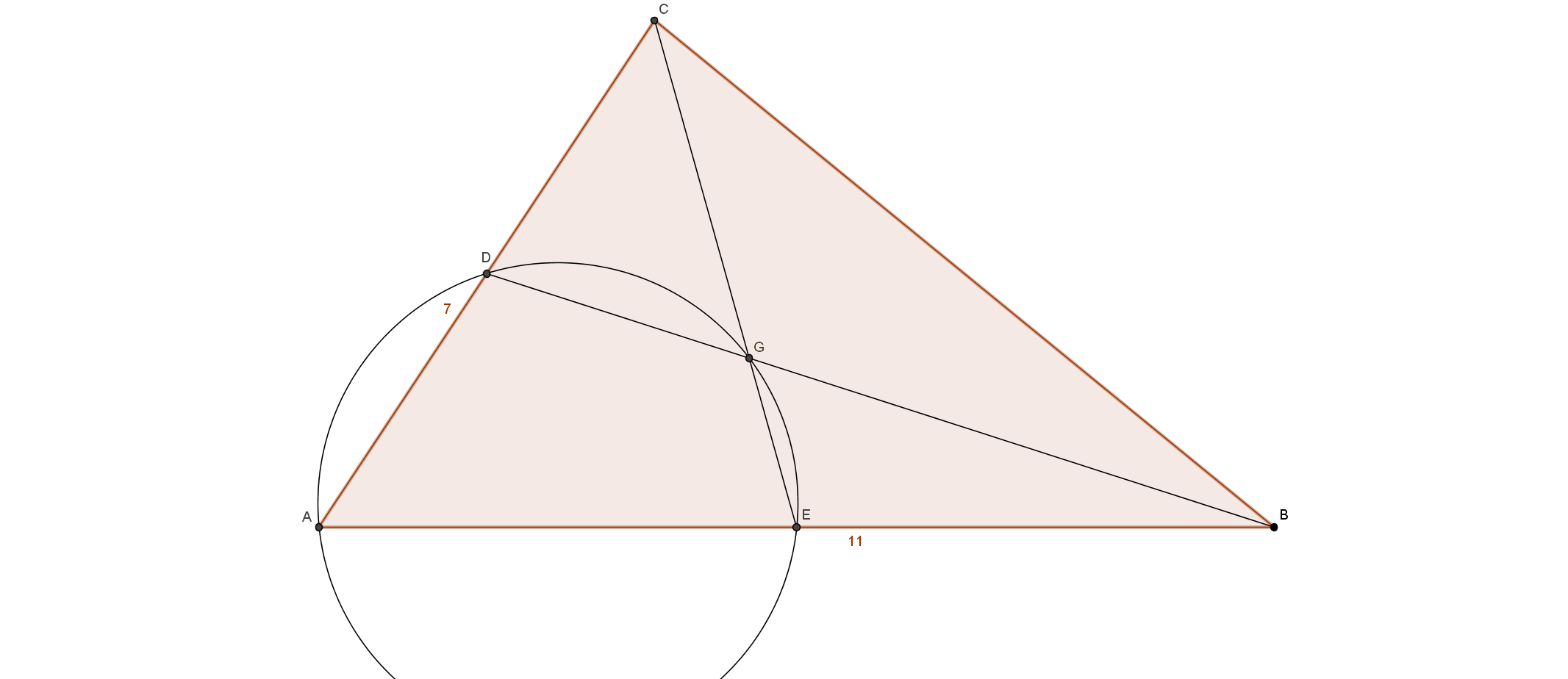# Centroid Circle

Geometry Level 5Let $ABC$ be a triangle, with $AB=11$ and $AC=7$, and let $D,E,G$ respectively be the midpoint of the side $AC$, the midpoint of $AB$ and the centroid of $ABC$. Knowing that $A,D,E,G$ are concyclic, and that $BC=\sqrt{n}$, find $n$.

×

Problem Loading...

Note Loading...

Set Loading...